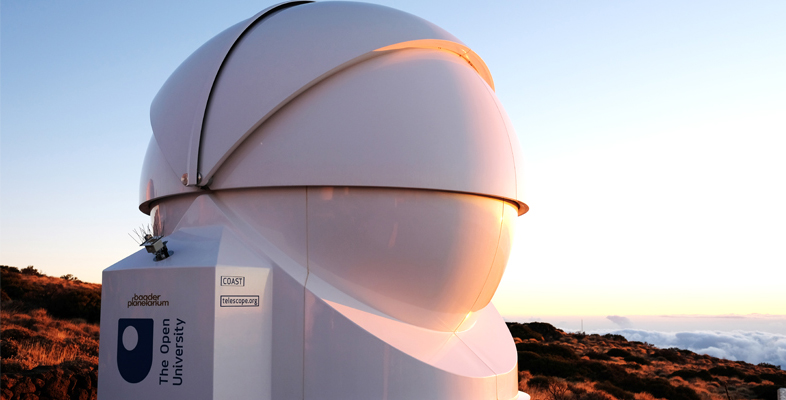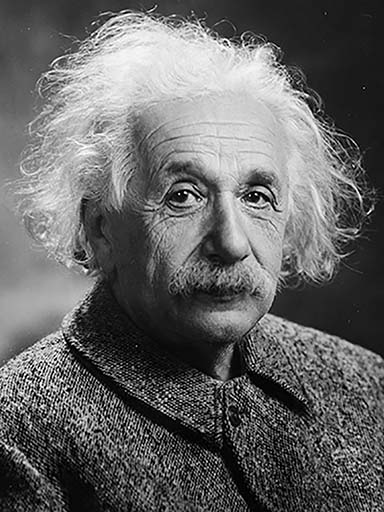Science, Maths & Technology

### Become an OU studentAstronomy with an online telescope

Start this free course now. Just create an account and sign in. Enrol and complete the course for a free statement of participation or digital badge if available.

# 3.1 The most famous equation in the worldFigure 6 Albert Einstein, who in 1905 formulated the theory of relativity.

Perhaps the most famous and well-known equation in the whole of physics is Albert Einstein’s .

This simple-looking equation holds the key to the source of the Sun’s energy. The equation is a direct consequence of Einstein’s theory of relativity and essentially states that energy and mass are equivalent and that under certain circumstances, such as during nuclear reactions, an amount of matter can be converted into energy.

The other term in the equation, , sets an exchange rate for this conversion. The here is the speed of light: at 300 000 km per second this is already a very large number and in Einstein’s equation it is squared – multiplied by itself – making a vast number. This huge conversion factor means that a small amount of mass is equivalent to an extremely large amount of energy.

As a result of this conversion factor, nuclear reactions are immensely more powerful than chemical reactions. The Drax power station that you saw in Section 2 consumes over 9 million tonnes of coal every year, yet nuclear power stations such as Sizewell B run on just 30 to 40 tonnes of nuclear fuel. Even in nuclear reactions, only a small fraction of the mass of the fuel is converted into energy using . Of the 30 to 40 tonnes of nuclear fuel used at Sizewell every year, just over one kilogram of that mass is converted to energy.